Practice the AP 10th Class Physical Science Bits with Answers Chapter 10 Electromagnetism on a regular basis so that you can attempt exams with utmost confidence.

## AP State Syllabus 10th Class Physical Science Bits 10th Lesson Electromagnetism with Answers

Question 1.
The unit of magnetic field strength is
A) Oersted
B) Ampere
C) Coloumb
A) OerstedQuestion 2.
The magnetic field around a magnet is
A) one dimensional
B) two dimensional
C) three dimensional
D) none
C) three dimensional

Question 3.
Which of the following statement is true ?
A) A magnetic field is characterized by strength and direction.
B) The direction of the magnetic field can be determined by using a compass.
C) The strength of the magnetic field varies with distance from the bar magnet.
D) all of the above
D) all of the above

Question 4.
Statement X : The magnetic field is strong when lines are crowded.
StatementY : The magnetic field is weak when lines are spaced apart.
A) Both statements are true
B) Both statements are false
C) X is true, Y is false
D) X is false, Y is true
A) Both statements are true

Question 5.
Statement X : If the field strength or direction of field change from point to point then the field is said to be non-uniform.
StatementY : If both field strength and direction of field are constant throughout the field then the field, is said to be uniform.
A) Both statements are true
B) Both statements are false
C) X is true, Y is false
D) X is false, Y is true
A) Both statements are true

Question 6.
Which of the following statements is correct ?
A) The magnetic field produced by a bar magnet-is non-uniform.
B) The magnetic field produced by a bar magnet is uniform.
C) Earth’s magnetic field is uniform.
D) Earth’s magnetic field is non¬uniform.
C) Earth’s magnetic field is uniform.

Question 7.
The number of lines passing through the plane of area perpendicular to the field is called
A) magnetic field
B) magnetic flux
C) magnetic flux density
D) none
B) magnetic flux

Question 8.
The magnetic flux passing through unit area taken perpendicular to the field is called …………………
A) magnetic flux
B) magnetic flux density
C) magnetic field induction
D) both B and C
D) both B and CQuestion 9.
The ratio of magnetic flux passing through a plane perpendicular to the field and area of the plane is called …………….
A) magnetic flux
B) magnetic flux density
C) magnetic field induction
D) both B and C
D) both B and C

Question 10.
The S.I unit of magnetic flux is ………………..
A) Weber
B) Weber/meter2
C) Tesla
D) Both B and C
A) Weber

Question 11.
The S.I unit of magnetic flux density is ………………….
A) Weber
B) Weber/meter2
C) Telsa
D) Both B and C
D) Both B and C

Question 12.
Statement X : Magnetic flux represents the number of lines passing through the plane in the field.
Statement Y : The magnetic flux depends on the orientation of the plane in the field.
A) Both statements are true
B) Both statements are false
C) X is true, Y is false.
D) X is false, Y is true
A) Both statements are true

Question 13.
The general formula of the flux for any orientation of the plane taken in the field
A) Φ = BA
B) Φ = BA cos θ
C) Φ = BA sin θ
D) zero
B) Φ = BA cos θ

Question 14.
The flux through the plane taken perpendicular to the field is……………….
A) Φ = BA
A) Φ = BA
B) Φ = BA cos θ
C) Φ = BA sin θ
D) zero
A) Φ = BA

Question 15.
The flux through the plane taken parallel to the field is……………….
A) Φ = BA
A) Φ = BA
B) Φ = BA cos θ
C) Φ = BA sin θ
D) zero
D) zero

Question 16.
The scientist who discovered the magnetic effect of current:
A) Oersted
C) Ampere
D) Fleming
A) Oersted

Question 17.
The shape of the magnetic field lines around a straight current carrying conductor are …………….
A) parallel lines
B) concentric circles
C) both A and B
D) none
B) concentric circles

Question 18.
Statement X: When electric current flows vertically upwards the direction of the magnetic field lines produced by a straight conductor are in anti clock wise direction.
Statement Y : When electric current flows vertically down-wards the direction of the magnetic field lines produced by a straight conductor are in clock wise direction.
A) Both statements are true
B) Both statements are false
C) X is true, Y is false
D) X is false, Y is true
A) Both statements are trueQuestion 19.
Which of the following diagram represents the direction of magnetic field lines produced by a straight conductor when an electric currentflows in up ward direction i.e out of the page?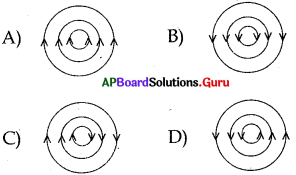D

Question 20.
Statement X:
The direction of the magnetic field due to circular coil points to wards you when the current in the coil is in anti clock wise direction.
Statement Y : The direction of the magnetic field due to circular coil points away from you when the current in the coil is . clock wise direction.
A) Both statements are true
B) Both statements are false
C) X is true, Y is false
D) X is false, Y is true
A) Both statements are true

Question 21.
Which of the following statements is correct ?
A) A current carrying circular wire behaves like a thin disc magnet
B) If the current flows around the face of a circular coil in the clock-wise direction that face of the circular wire will be south pole.
C) If the current flows around the face of a circular coil in the anti clock-wise direction that face of the circular wire will be north pole.
D) All of the above
D) All of the above

Question 22.
The direction of current flowing in a coil is shown in figure. What type of magnetic pole is formed at the face?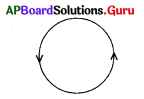A) North pole
B) South pole
C) East pole
D) West pole
A) North pole

Question 23.
Which of the following statements is, are correct?
A) A long wire wound in a closely packed helix is called solenoid.
B) The magnetic field lines set up by solenoid resemble those of a bar magnet.
C) One end of the solenoid behaves like a north pole and other behaves like a south pole when electric current flows through it.
D) All of the above
D) All of the above

Question 24.
Statement X : The direction of the field’ outside the solenoid is from north to south.
Statement Y : The direction of the field lines inside the solenoid is from south to north.
A) Both statements are true
B) Both statements are false
C) X is true, Y is false
D) X is false, Y is true
A) Both statements are true

Question 25.
Statement X : The strength of the magnetic field inside a solenoid is the same at all the points.
Statement Y : The strength of the magnetic field is the same at all points outside the solenoid.
A) Both statements are true
B) Both statements are false
C) X is true, Y is false
D) X is false, Y is true
C) X is true, Y is false

Question 26.
Which of the following statements is aretrue ?
A) The direction of the magnetic field lines due to straight current carrying wire can be determined by using right hand rule.
B) The direction of the magnetic field lines due to circular current carrying wire can be determined by using right hand rule.
C) The direction of the magnetic field lines due to current carrying solenoid can be determined by using right hand rule.
D) All of the above
D) All of the aboveQuestion 27.
For the solenoid given in the diagram name the polarities A and B of a solenoid when the current flows in the direction
shown.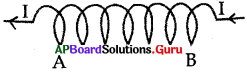A) North and south
B) South and north
C) East and west
D) West and east
A) North and south

Question 28.
Tesla is the S.I unit for ………………….
A) magnetic flux
B) magnetic flux density
C) magnetic pole strength
D) induction emf
B) magnetic flux density

Question 29.
The direction of lines of force in the inner side of a solenoid is ………………….
A) North to south
B) South to north
C) East to west
D) West to east
B) South to north

Question 30.
The magnetic field is called uniform if
A) strength and direction of magnetic field are changing
B) strength and direction of magnetic field are constant
C) only magnetic field strength is constant
D) only direction of magnetic field is constant
B) strength and direction of magnetic field are constant

Question 31.
Magnetic flux density (B) = ……………..
A) Area/Magnetic flux
B) Length/Magnetic flux
C) Magnetic flux/Length
D) Magnetic flux/ Area
D) Magnetic flux/ Area

Question 32.
If B is the magnetic flux density and A is the area of the plane then the magnetic flux is given by
A) AB
B) B/A
C) A/B
D) A2B
A) AB

Question 33.
A current carrying circular coil has ‘n’ turns. The magnetic field produced in the entire coil is
A) 1/n times as large as that produced by a single turn
B) same as that produced by a single turn
C) ‘n’ times as large as that produced by a single turn
D) zero
C) ‘n’ times as large as that produced by a single turn

Question 34.
The first scientist who identified the relationship between electricity and magnetism is ………………..
A) Oersted
B) Ampere
C) Lenz
D) Ohm
A) Oersted

Question 35.
1 tesla = ………………..
A) 1 Weber
B) 1 Weber/meter2
C) 1 Watt/meter2
D) 1 Coloumb
B) 1 Weber/meter2

CONCEPT – II: Magnetic Force on Moving Charge and Current Carrying Wire – Electric Motor

Question 36.
The equation for magnetic force on charge q when there is an angle “9” between the direction of field B and
velocity V is ………………….
A) F = Bqv
B) F = Bqv sinθ
C) F = Bqv cosθ
D) zero
B) F = Bqv sinθ

Question 37.
The equation for magnetic force on charge ‘q’ moving perpendicular to the magnetic field is
A) F = Bqv
B) F = Bqv sinθ
C) F = Bqv cosθ
D) zero
A) F = BqvQuestion 38.
The magnetic force on the charge ‘q’ moving parallel to the magnetic field is ……………………
A) F = Bqv
B) F = Bqv sinθ
C) F = Bqv cosθ
D) zero
D) zero

Question 39.
Statement X : The picture on the TV screen is distorted when you bring a bar magnet near the TV screen as the magnetic field exerts a force on the moving charges.
Statement Y : When you bring a bar magnet away from the TV screen you will get a clear picture on the screen because the force exerted by the magnet on the charges disapp-ear.
A) Both statements are true
B) Both statements are false
C) X is true, Y is false
D) X is false, Y is true
A) Both statements are true

Question 40.
The equation for magnetic force on a current carrying wire of length T/ which makes an angle ‘θ’ with the magnetic
field is ……………….
A) F = BIL
B) F = BIL sinθ
C) F = BIL cosθ
D) zero
B) F = BIL sinθ

Question 41.
The equation for magnetic force on a current carrying wire of length T/ which is placed perpendicular to a magnetic field is ………………….
A) F = BIL
B) F = BIL sinθ
C) F = BIL cosθ
D) zero
A) F = BIL

Question 42.
The magnetic force on a current carrying wire which is placed along a magnetic field is …………..
A) F = BIL
B) F = BIL sinθ
C) F = BIL cosθ
D) zero
D) zero

Question 43.
In which way does the wire move which is placed perpendicular to the magnetic field and the direction of current is Shown in figure? [The direction of the magnetic field is into the page.i.e x]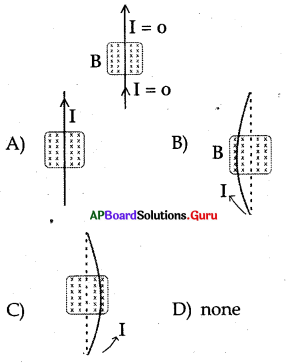B

Question 44.
A current carrying wire of length L is placed perpendicular to a uniform magnetic field B. Then the force acting on the wire with current is ……………………..
A) 0
B) ILB
C) 2ILB
D) ILB/2
B) ILB

Question 45.
The charge is moving alongthe direction of magnetic field. Then force acting on it is ………………..
A) 0
B) Bqv sinθ
C) Bqv
D) BIL
A) 0

Question 46.
Name the device which is used to reverse the direction of current in the coil of a
A) commutator
B) Split rings
C) both A and B
D) none
C) both A and B

Question 47.
In an electric motor which of the following rotates with the coil ?
A) commutator
B) brush
C) both A and B
D) none
A) commutatorQuestion 48.
In an electric motor which of the following remains fixed with the coil ?
A) commutator
B) brush
C) both A and B
D) none
B) brush

Question 49.
In an electric motor the direction of current in the coil charge once in each ………………
A) two rotations
B) one rotation
C) half – rotation
D) one – fourth – rotation
C) half – rotation

Question 50.
Statement X : A current carrying coil rotates when it is kept in a uniform magnetic field.
Statement Y: Net force on a current carrying coil when it is kept in a uniform magnetic field is zero.
A) Both statements are true
B) Both statements are false
C) X is true, Y is false
D) X is false, Y is true
A) Both statements are true

Question 51.
Which of the following statements is correct.
A) The direction of rotation of motor will be reversed when the direction of the current is reversed.
B) The direction of the rotation of motor will be reversed when the direction of the magnetic field is reversed.
C) The direction of the rotation of motor will remain unchanged when both current and magnetic field are reversed simultaneously.
D) AH of the above
D) AH of the above

Question 52.
Statement X : When a current carrying wire is kept perpendi-cular to the magnetic field it experiences a force.
Statement Y : When a current carrying wire is kept along the direction (or) against the direction of magnetic field it experiences no force.
A) Both statements are true
B) Both statements are false
C) X is true, Y is false
D) X is false, Y is true
A) Both statements are true

Question 53.
As shown in the figure a positive charge ‘q’ moves at a speed ‘v’ in a constant uniform magnetic field directed into the page. The direction of velocity is perpendicular to the direction of magnetic field. The direction of magnetic force on the charge ‘q’ is …………………
A) due north
B) due south
C) due west
D) due east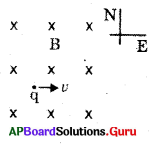A) due north

Question 54.
Which .of the following equation is correct in electro magnetism ?
A) F = $$\frac{\mathrm{qv}}{\mathrm{B} \sin \theta}$$
B) F = $$\frac{\mathrm{qB} \sin \theta}{\mathrm{v}}$$
C) F = qvBsinθ
D) F = qvBcosθ
C) F = qvBsinθ

Question 55.
In which of the following electric energy is converted into mechanical energy ?
A) Galvanometer
B) Ammeter
C) Generator
D) Electric motor
D) Electric motor

Question 56.
A particle of charge ‘q’ and rhass’m’ is moving with a speed V perpendicular to the magnetic field of induction B. The radius of the circular path moving by the particle is ………………….
A) mvB/q
B) mlvq/B
C) mB/vq
D) mv/Bq
D) mv/Bq

Question 57.
If the angle between moving charge and magnetic field is 0, the magnetic force acting on the charge is given by …………….
A) qvB $$\frac{1}{\operatorname{cosec} \theta}$$
B) qvBcosθ
C) qvB. $$\frac{1}{\sin \theta}$$
D) qvB tanθ
A) qvB $$\frac{1}{\operatorname{cosec} \theta}$$Question 58.
No force works on the conductor carrying electric current when it is kept ………………….
A) away from magnetic field
B) in the magnetic field
C) perpendicular to magnetic field
D) parallel to magnetic field
D) parallel to magnetic field

Question 59.
A charge ‘q’ is moving with a velocity ‘v’ in magnetic field of induction B. If the magnetic force acting on charge ‘q’ is equal to qvB, then ………………….
A) ‘q’ is moving parallel to B
B) ‘q’ is moving perpendicular to B
C) ‘q’ is moving at an angle of 45° to B
D) ‘q’ is stationary
B) ‘q’ is moving perpendicular to B

Question 60.
If we stretch the thumb, index finger and middle finger perpendicular to each other in the right hand rule with regard to positive charge then these three fingers indicate the following direction respectively (when direction force is ‘F’, direction of velocity of charge is V, direction of field is ‘B’)
A) F, V, B
B) V, F, B
C) B,V,F
D) F, B, V
A) F, V, B

CONCEPT – III: Electro – Magnetic Induction

Question 61.
The phenomenon of electro magnetic induction is ………………….
A) the process of charging a body.
B) the process of generating magnetic field due to current passing through a coil
C) producing induced current in a coil due to relative motion between a magnet and a coil
D) the process of rotating a coil of electic motor
C) producing induced current in a coil due to relative motion between a magnet and a coil

Question 62.
Statement X : Whenever there is a continuous charge of magnetic flux linked with a closed coil a current is generated in the coil. This is Faraday’s law of electic magnetic induction.
Statement Y : The induced emf generated in a closed loop is equal to the rate of charge of magnetic flux passing through it. This is Faraday’s law of electro magnetic indue – tion.
A) Both statements are true
B) Both statements are false
C) X is true, Y is false
D) X is false, Y is true
A) Both statements are true

Question 63.
The equation of Faraday’s law of electro magnetic induction is
A) G = Δt / ΔΦ
B) e = ΔΦ/Δt
C) e = ΔΦx Δt
D) none
B) e = ΔΦ/Δt

Question 64.
Faraday’s law of induction is the consequence of …………….
A) law of conservation of mass
B) law of conservation of charge
C) law of conservation of energy
D) none
C) law of conservation of energy

Question 65.
Statement X : Lenz’s law states “the induced current will appear in such a direction that it opposes the charges in the flux in the coil.”
Statement Y : Lenz’s law gives the direction of induced curren in a coil
A) Both statements are true
B) Both statements are false
C) X is true, Y is false
D) X is false, Y is true
A) Both statements are true

Question 66.
Find the length of the conductor which is moving with a speed of 10 m/sin the direction perpendicular to the direction of magnetic field of induction 0.8T, if it induces an em/of 8V between the ends of the conductor.
A) 10 m
B) 1 m
C) 100 m
D) 0.8 m
B) 1 m

Question 67.
Which of the following works on the rule of Faraday’s law of electro -magnetic induction ?
A) Tape recorder
B) ATM machine
C) Induction stove
D) All
D) All

Question 68.
The frequency of direct current is …………………
A) 0 Hz
B) 50 Hz
C) 60 Hz
D) 100 Hz
A) 0 Hz

Question 69.
The frequency of alternating current (AC) supply in India is ……………..
A) 0Hz
B) 50 Hz
C) 60 Hz
D) 100 Hz
B) 50 Hz

Question 70.
Statement X : Induced emf is genera¬ted due to relative motion between the magnet and the coil
Statement Y : Induced emf is genera¬ted due to continuous charge of magnetic flux linked with a closed coil (AS 2) ( )
A) Both statements are true
B) Both statements are false
C) X is true, Y is false
D) X is false, Y is true
A) Both statements are trueQuestion 71.
The essential difference between an AC generator and a DC generator is that
A) AC generator has an elector magnet while a DC generator has permanent magnet
B) DC generator will generate a higher voltage
C) AC generator has slip rings while the DC generator has a commutator.
D) AC generator has commutator while the DC generator has slip rings
C) AC generator has slip rings while the DC generator has a commutator.

Question 72.
Statement X : A generator works on the principle of electro megnetic induction.
Statement Y : A motor works on the principle of electro magnetic induction.
A) Both statements are true
B) Both statements are false
C) X is true, Y is false
D) X is false, Y is true
C) X is true, Y is false

Question 73.
The given figure represents .
A) DC
B) AC
C) both A and B
D) none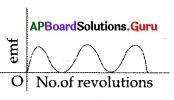A) DC

Question 74.
The given figure represents
A) DC
B) AC
C) both A and B
D) none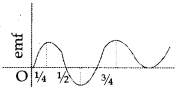B) AC

Question 75.
Which of the following does not work on electro magnetic induction principle
A) Transformer
B) Tape recorder
C) ATM card
D) None
D) None

Question 76.
Whenever there is continuous changes of magnetic flux linked with closed coil a current is generated in coil. This is ……………..
A) Lenz’s law
B) Ampere’s law
D) Ohm’s law

Question 77.
A conductor of length of 1m moving with velocity ‘v’ perpendicular to the magnetic field of strength 10 Tesla induces an e.m.f of 10 volts. Then v = ………….. m/s
A) 100
B) 10
C) 1
D) 0.1
C) 1

Question 78.
The peak value of alternative current is 2 ampere. The r.m.s value of current is ………….. ampere.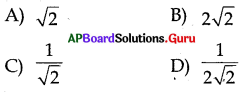B

Question 79.
Which among the following is responsible for an induced current in the coil ?
A) Presense of galvanometer in the coil
B) Presense of magnetic flux in the coil
C) Change of magnetic flux through the coil
D) Using coil of large area of cross – section
C) Change of magnetic flux through the coil

Question 80.
A coil has 200 turns. If an increase in , magnetic flux through one turn of coil is 0.001 Wb in 0.1 sec, then the maximum induced emf in the coil is ………….
A) 0.2 V
B) 2 V
C) 20 V
D) 20000 V
B) 2 V

Question 81.
A metal ring is inserted through the soft iron cylinder which is wounded with copper wire when AC is supplied between the ends of the coil, then ……………..
A) the metal ring is levitated on the coil.
B) the metal ring is levitated and falls down immediately.
C) the metal ring rotates round the cylinder without levitation.
D) none.
A) the metal ring is levitated on the coil.

Question 82.
Generator converts mechanical energy to ……………. energy.
A) sound
B) light
C) electrical
D) wind
C) electricalQuestion 83.
…………………. generated in a closed loop is equal to the rate of change of magnetic flux passing through it.
A) Motional emf BD
B) Induced emf
C) Motional resistance
D) Induced resistance
B) Induced emf

Question 84.
According to Faraday’s law the induced emf produced in a closed loop is equal to the …………….
A) magnetic flux
B) change of magnetic flux
C) rate of change of magnetic flux
D) cross – sectional area of the loop.
C) rate of change of magnetic flux

Question 85.
A conductor is moving with a speed of 10 nVs perpendicular to the direction of magnetic field of induction 0.5 T. If the induced emf is 5 V then the length of the conductor is …………………….
A) 0.25 m
B) 0.01 m
C) 4 m
D) 1 m
D) 1 m

Question 86.
A metal ring is inserted through the soft iron cylinder which is wounded with copper wire. When DC is supplied between the ends of the coil, then …………………….
A) the metal ring is levitated on the coil and stays there.
B) the metal ring is levitated and falls down immediately.
C) the metal ring rotate round the cylinder at the same position.
D) none
B) the metal ring is levitated and falls down immediately.

Question 87.
A conductor of 1 m length is moving with a speed of 10 m/s in the direction perpendicular to the direction of magnetic field of induction 0.8T. The induced e.m.f between the ends of the conductor is …………………….
A) 10 V
B) 1 V
C) 2 V
D) 8 V
D) 8 V

Question 88.
An induction stove works on this principle
A) electric induction
B) induced emf
C) electro magnetic induction
D) magnetic induction.
C) electro magnetic induction

Question 89.
The law which states “An induced emf will appear in such a direction that it opposes the change in its flux” is …………………….
B) Kirchhoff’s loop law
C) Ohm’s law
D) Lenz’s law
D) Lenz’s law

Question 90.
In which among the following the principle of electromagnetic induction is not involved ?
A) In security check, where people are made to walk through a large upright coil of wire
B) Working of tape recorder
C) Working of an electric bulb
D) Working of ATM cards.
C) Working of an electric bulb

Question 91.
If ΔΦ/Δt is the rate of change of flux and I is the current then the electric power is …………….
A) I. (Δt/ΔΦ)
B) I. (ΔΦ/Δt)
C) I2. (ΔΦ/Δt)
D) (ΔΦ/Δt)
B) I. (ΔΦ/Δt)

Question 92.
If I0 and V0 are the peak values of AC and AC – emf for an AC generator, then
their rms values respectively are …………….
A) I0/2,V0/2
B) $$\left(\mathrm{I}_{0} / \sqrt{2}, \mathrm{~V}_{0} / \sqrt{2}\right)$$
C) $$\mathrm{I}_{0} \cdot \sqrt{2}, \mathrm{~V}_{0} \cdot \sqrt{2}$$
D) 2I0,2V0
\left(\mathrm{I}_{0} / \sqrt{2}, \mathrm{~V}_{0} / \sqrt{2}\right)

Question 93.
…………….. current has some frequency
A) A.C
B) D.C
C) A.C & D.C
D) none
A) A.C

Question 94.
Electro magnetic induction was proposed by …………….
A) Oersted
C) Lenz
D) WeberQuestion 95.
The rms value of current is 110$$\sqrt{2}$$ A then its peak value of current is ………………
A) 220 A
B) 110 A
C) 110$$\sqrt{2}$$ A
D) 1104/$$\sqrt{2}$$ A
A) 220 A

Question 96.
The device used for producing electric current is ……………..
A) generator
B) galvanometer
C) ammeter
D) motor
A) generator

Question 97.
Statement X : DC generator uses split rings.
Statement Y : AC generator uses slip rings.
A) Both statements are true
B) Both statements are false
C) X is true, Y is false
D) X is false, Y is true